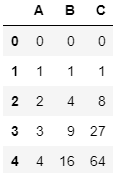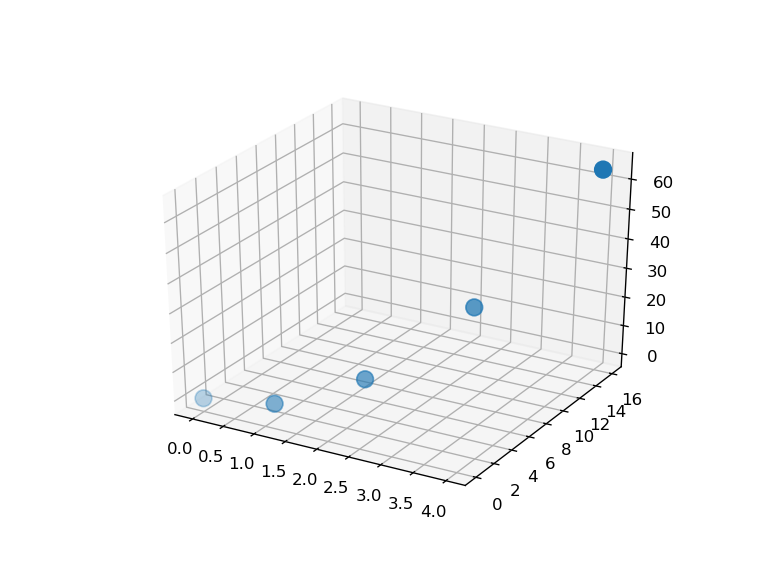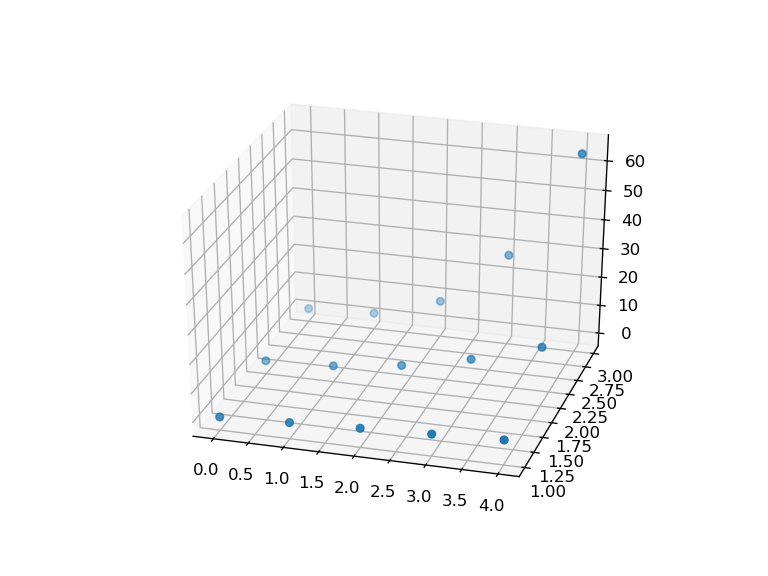# [PYTHON] 3D plot Pandas DataFrame

In my research, I wanted to plot Pandas DataFrame in 3D. For example, suppose you have the following data:It's very easy and full of articles to plot this data with A on the x-axis, B on the y-axis, and C on the z-axis, for example with the following code:

``````import pandas as pd
%matplotlib notebook
import matplotlib.pyplot as plt
from mpl_toolkits.mplot3d import Axes3D

df = pd.DataFrame({'A':[0,1,2,3,4],
'B':[0,1,4,9,16],
'C':[0,1,8,27,64]})
fig = plt.figure()
sc = ax.scatter(df.A, df.B, df.C, s=100)
``````You can plot it like this image.

Now, as for the main subject, I wanted to make a total of 15 3D plots with this data on the x-axis, index on the y-axis, and each data on the z-axis. Conclusion I could do it with the code below, but it was a bit annoying.

``````import pandas as pd
%matplotlib notebook
import matplotlib.pyplot as plt
from mpl_toolkits.mplot3d import Axes3D
import numpy as np

df = pd.DataFrame({'A':[0,1,2,3,4],
'B':[0,1,4,9,16],
'C':[0,1,8,27,64]})

#Since it is not possible to plot if it is a character, change the column name to a number
df.rename(columns={'A':1, 'B':2, 'C':3}, inplace=True)

X = np.array([])
Y = np.array([])
Z = np.array([])

for i in range(df.index.size):
X = np.concatenate([X, np.full(df.columns.size, df.index[i])], 0)

for i in range(df.index.size):
Y = np.concatenate([Y, np.array(df.columns)], 0)

for i in range(df.index.size):
Z = np.concatenate([Z, np.array(df[i:i+1])], 0)

fig = plt.figure()
It looks like the image below.Thank you for your hard work. I hope it helps someone.